# Var vs Dynamic in C#

## Var vs. Dynamic in C# with Examples

In this article, I am going to discuss Var vs. Dynamic in C# with Examples. Before proceeding with this article, I strongly recommend you read our Dynamic Keyword in C# and VAR Keyword in C# articles. At the end of this article, you will understand the differences between VAR and Dynamic, when to use VAR, and when to use Dynamic in C# with Examples.

##### Var vs. Dynamic in C#

In simple words, we can say that var is early bounded (in other words, it is statically checked) whereas Dynamic is late bounded (in other words, it is checked at runtime), or you can say it is dynamically evaluated. Let us understand the differences between the Var vs. Dynamic Keywords in C# with Examples.

Please have a look at the below example. Here, I have declared one variable, x, using the var keyword and assigned the value string1. Then I declared one integer variable Len to hold the length of the x variable. Here, I am calling the Length property on the x object.

```namespace VarVSDynamicDemo
{
class Program
{
static void Main(string[] args)
{
var x = "String1"; //Early Bounded
int Len = x.Length;
}
}
}
```

The first thing that you need to notice here is that when we type x and dot (.), you will notice that intelligence is coming and you can see the Length property as shown in the below image.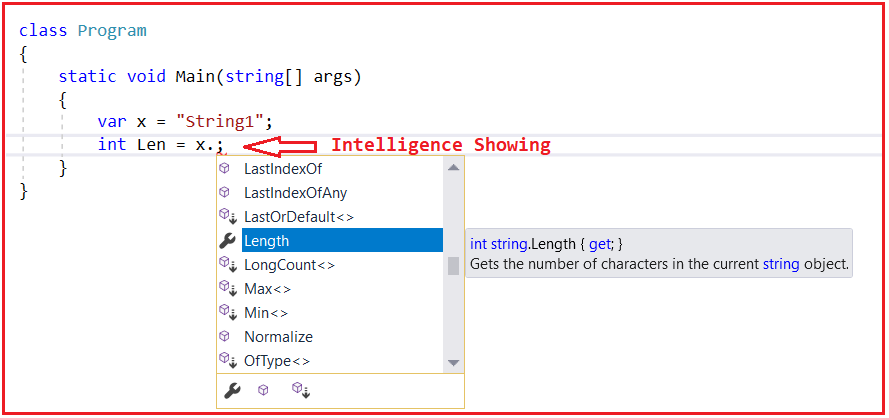And if you move the mouse pointer over the x variable, you will see that it is saying x is a local variable whose data type is a string, as shown in the image below.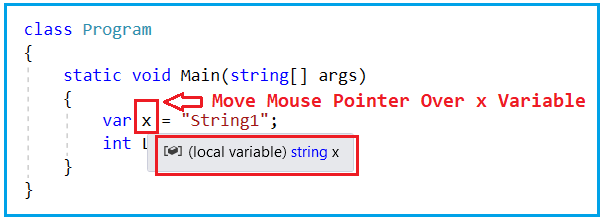So, in other words, the compiler figures out the x variable data type is a string at the time of compilation. In this case, the compiler looks at the right-hand side data (i.e., string1) and figures out the x data type as a string, as the right-hand side data is a string data. For a better understanding, please have a look at the below image.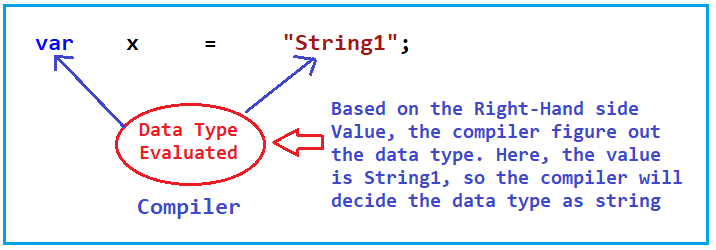The Var keyword is early bounded or statically checking. It means at the time we write the code using the var keyword and compile it; the compiler knows what the data type is.

Let us do the same thing using dynamic type in C#. The following is the same example as the previous one, except here, we use the dynamic keyword instead of the var keyword. So, here, I have declared one variable called x using the dynamic keyword and assigned the value string1. Then I declared one integer variable Len to hold the length of the x variable. Here, I am calling the Length property on the x object.

```namespace VarVSDynamicDemo
{
class Program
{
static void Main(string[] args)
{
dynamic x = "String1"; //Late Bounded
int Len = x.Length;
}
}
}
```

The first thing you need to notice here is that when we type x and dot (.), we will not get any intelligence, and we cannot see the Length property or any other properties or methods, as shown in the image below.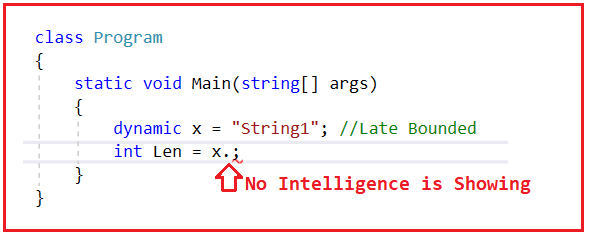And if you move the mouse pointer over the x variable, then you will see that it is saying that x is a local variable whose data type is dynamic, as shown in the below image. That means it is still not figuring out what is the data type of the variable x.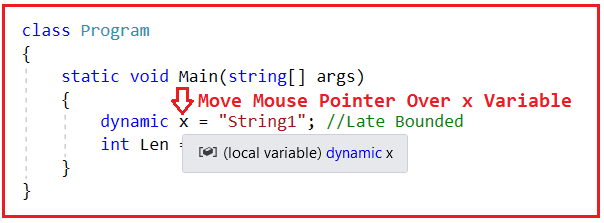##### Example to understand Var and Dynamic Keywords in C#:

Now, let us execute the following code in debug mode.

```using System;
namespace VarVSDynamicDemo
{
class Program
{
static void Main(string[] args)
{
var v = "String1"; //Late Bounded
int Len1 = v.Length;
Console.WriteLine(\$"Using Var, Value={v} and Length={Len1}");

dynamic d = "String1"; //Late Bounded
int Len2 = d.Length;
Console.WriteLine(\$"Using Dynamic, Value={d} and Length={Len2}");

}
}
}
```

Executing the statements using the var keyword is straightforward. This is because property binding, i.e., invoking the Length property on the v object, is bounded at the compilation time. This is because the compiler knows a property called Length is available in the string class. But this is not the case with dynamic type. So, what happens with dynamic type is, at runtime, the d variable dynamically goes and uses reflection internally and tries to invoke the Length property dynamically. If the property exists, it will execute, and if it does not, it will throw a runtime exception. In our example, the Length property exists in the string class and will execute that property.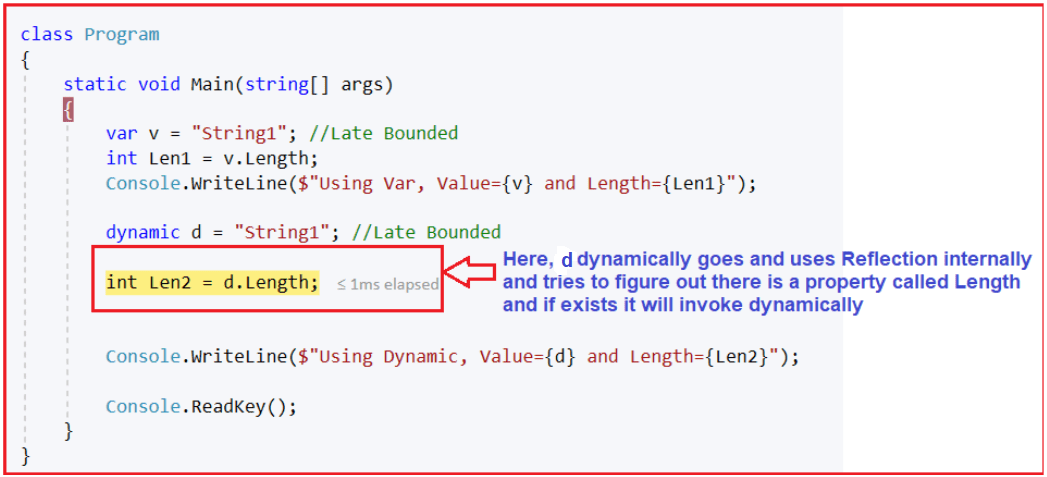So, when you execute the above code, you will get the following output as expected.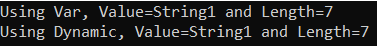Now, let us make a small mistake. Instead of Length (capital L), let us use length (small l) and see what happens. See, with the var keyword; we are getting compile time error immediately. But, with the dynamic keyword, we are not getting any compile time error. This is because the binding has not happened at the compile time. With dynamic, binding will going to happen at runtime only.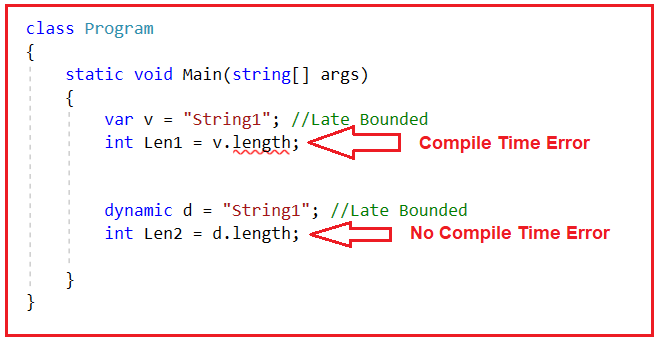Let us try to use capital L with the var keyword and small l with the dynamic keyword, as shown below, and try to run the application.

```using System;
namespace VarVSDynamicDemo
{
class Program
{
static void Main(string[] args)
{
var v = "String1"; //Late Bounded
int Len1 = v.Length;

dynamic d = "String1"; //Late Bounded
int Len2 = d.length;
}
}
}
```

You can observe that we are not getting any compilation errors. But when we run the code, we will get the following Runtime Exception. This is because, at runtime, d internally uses the Reflection mechanism to invoke the length property of the string class. But in the string class, there is no property length (with a small l), so it will throw a runtime error.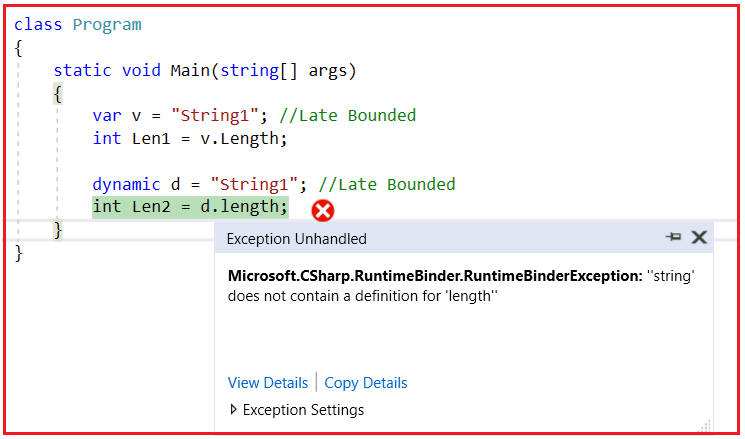So, the difference between var and dynamic keywords in C# is that var is Early Bounded (it is statically checked, or you can say it is checked at compilation time), whereas dynamic is Late Bounded (the methods, the properties, the type, everything will be checked at runtime only).

##### Can we declare Properties using Var and Dynamic in C#?

In C#, we can use dynamic to declare properties, but we cannot use var. This is because, as we already discussed var can only be used to declare local variables inside a function, and properties are class members. And hence we cannot declare properties using the var keyword in C#.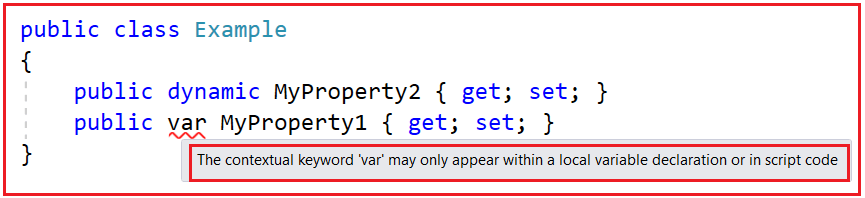Note: The most important point that you need to remember is dynamic keyword uses Reflection behind the scene. With this kept in mind, let us proceed and see what are the things we can and can’t do with var and dynamic keywords in C#.

##### Can we Change the Type of value after being assigned in Var and Dynamic in C#?

var does not allow the type of value assigned to be changed. That means if we assign an integer value to a var variable, we cannot assign a string or another type of value. This is because var will be treated as an integer type once we assign the integer value. And hence, thereafter, it will not allow other types of values. For a better understanding, please have a look at the following example.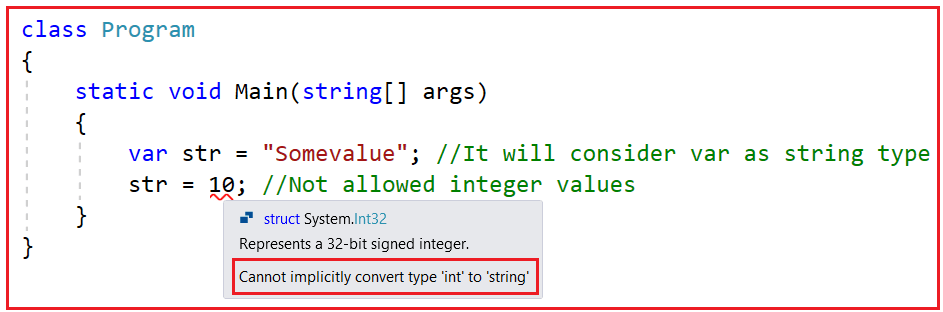But this is possible with dynamic type in C#. dynamic type allows the type of value assigned to be changed. That means if we assign an integer value to a var variable, we can also assign a string value or another type of value. This is because the type of variable will be decided at runtime, and based on the right-hand side value, the type will also be changed. For a better understanding, please have a look at the following example. This is the same example as the previous one, and here we are not getting any compilation error as well as we are not getting any runtime error.

```using System;
namespace VarVSDynamicDemo
{
class Program
{
static void Main(string[] args)
{
dynamic str = "Somevalue"; //It will consider str as string type at Runtime
Console.WriteLine(str.GetType());
str = 10; //str type changed from string to int type at Runtime
Console.WriteLine(str.GetType());
}
}
}```

System.String
System.Int32

##### Can we use var and dynamic as a function’s return type or parameter in C#?

We can not use the var keyword either as a function’s return type or a function or a parameter of a function in C#. The var keyword in C# can only be used as a local variable inside a function. If we try to use the var keyword as a method parameter or method return type, we will get a compiler time error.

For a better understanding, please have a look at the below example. Here, we are trying to use var as the return type of the SomeMethod as well as using var as the parameters of the SomeMethod.

```using System;
namespace VarVSDynamicDemo
{
class Program
{
static void Main(string[] args)
{
}

static var SomeMethod(var x, var y)
{
return x + y;
}
}
}
```

When you compile the above code, you will get the following compilation error.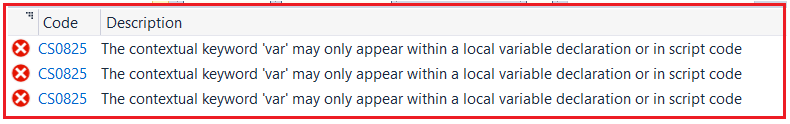As you can see, it is clearly saying that you can only use var as a local variable declaration. That means you cannot use var for either method return type or method parameter. Now, let us rewrite the same example using the dynamic keyword as follows. Here, in the below example, you can see that we are using dynamic as the return type of the SomeMethod and dynamic keyword to declare the method parameters of the SomeMethod.

```using System;
namespace VarVSDynamicDemo
{
class Program
{
static void Main(string[] args)
{
Console.WriteLine(SomeMethod(10, 20));
}

static dynamic SomeMethod(dynamic x, dynamic y)
{
return x + y;
}
}
}
```

Output: 30

Now, with the dynamic keyword in C#, we are not getting any compilation errors or runtime errors. That means we can use dynamic as a local variable, as the method return type, as well as the method parameter. This is one of the biggest differences between var and dynamic keywords in C#.

##### Differences Between Var and Dynamic in C#:

Now, let us summarizes the differences between var and dynamic in C#. The differences are as follows:

##### Var in C#
1. var is known as a statically typed variable, meaning that the data type of these variables is identified at compile time, which is done based on the type of value with which these variables are initialized.
2. var in C# was introduced as part of C# 3.0.
3. In the case of var, the variable’s data type is identified by the compiler at the compilation time only.
4. In the case of var, it is mandatory to initialize the variable at the time of its declaration so that the compiler comes to know the variable’s data type according to the right-hand side value assigned to it.
5. It will throw an error if the variable does not initialize at the time of its declaration.
6. We will get intelligence support in the visual studio.
7. Var cannot be used to declare method parameters and method return type in C#. It can only be used as a local variable declaration inside a function.
8. Var is early bounded. This means the compiler decides the type of variable declared at compile time.
##### Dynamic in C#
1. Dynamic is known as a dynamically typed variable which means that the data type of these variables is identified at runtime, which is done based on the type of value that these variables are initialized with.
2. Dynamic in C# was introduced in C#4.0.
3. In the case of dynamic, the data type of variable is identified by the CLR at run time.
4. In the case of dynamic, it is not mandatory to initialize the variable at the time of its declaration.
5. It will not throw an error if the variable does not initialize at the time of its declaration.
6. We will not get any intelligence support in the visual studio.
7. Dynamic can be used to declare method parameters and method return type in C#. It can also be used as a local variable declaration inside a function.
8. Dynamic is Late Bounded. This means the CLR decides the type of variable declared at runtime time.

1.